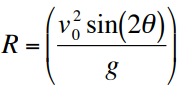The maximum horizontal range is obtained when _____.

1. $$\begin{array}{l}\Theta=45^{\circ}\end{array}$$
2. $$\begin{array}{l}\Theta=30^{\circ}\end{array}$$
3. $$\begin{array}{l}\Theta=90^{\circ}\end{array}$$
4. $$\begin{array}{l}\Theta=180^{\circ}\end{array}$$

$$\begin{array}{l}\Theta=45^{\circ}\end{array}$$

Explanation:

The horizontal range is given by the equationThe range is maximum when Sin 2θ is maximum, i.e., when θ=45o(2)(2)